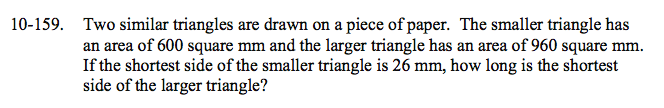Home > CCA2 > Chapter 10 > Lesson 10.3.1 > Problem10-159

10-159.

Two similar triangles are drawn on a piece of paper. The smaller triangle has an area of 600 square mm and the larger triangle has an area of 960 square mm. If the shortest side of the smaller triangle is 26 mm, how long is the shortest side of the larger triangle? Homework Help ✎$\text{If the ratio of the areas is }\frac{600}{960}, \text{ then the ratio of the sides is }\frac{\sqrt{600}}{\sqrt{960}}.$